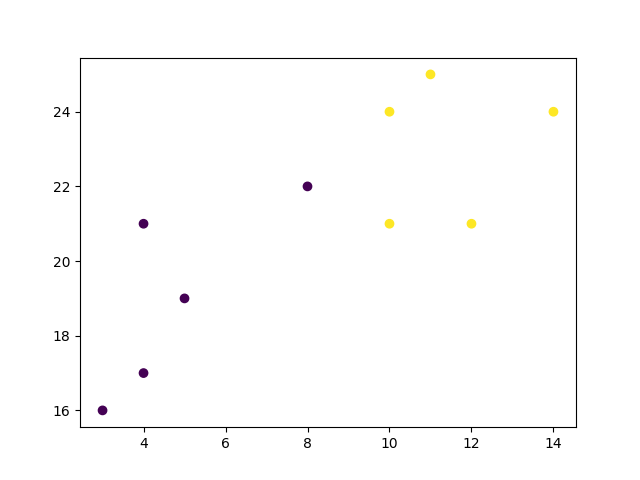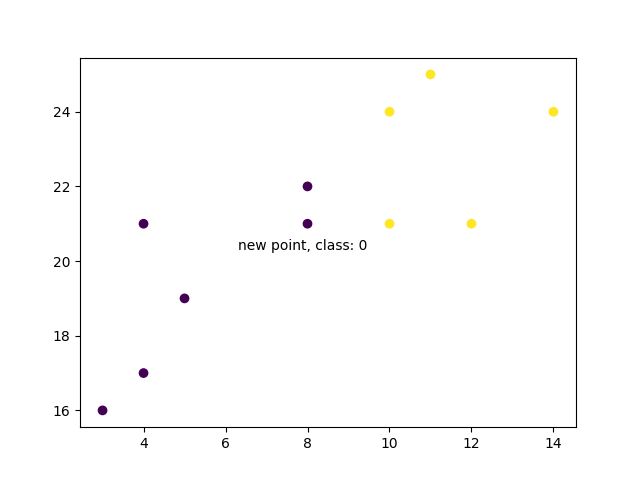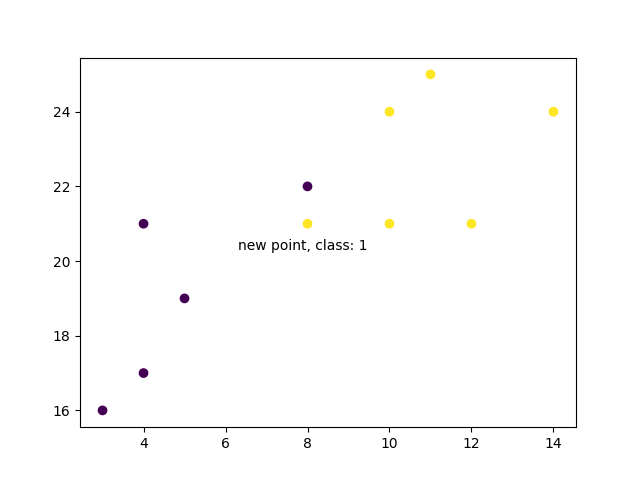## Python Tutorial

Python HOME Python Intro Python Get Started Python Syntax Python Comments Python Variables Python Data Types Python Numbers Python Casting Python Strings Python Booleans Python Operators Python Lists Python Tuples Python Sets Python Dictionaries Python If...Else Python While Loops Python For Loops Python Functions Python Lambda Python Arrays Python Classes/Objects Python Inheritance Python Iterators Python Scope Python Modules Python Dates Python Math Python JSON Python RegEx Python PIP Python Try...Except Python User Input Python String Formatting

## File Handling

Python File Handling Python Read Files Python Write/Create Files Python Delete Files

## Python Modules

NumPy Tutorial Pandas Tutorial SciPy Tutorial Django Tutorial

## Python Matplotlib

Matplotlib Intro Matplotlib Get Started Matplotlib Pyplot Matplotlib Plotting Matplotlib Markers Matplotlib Line Matplotlib Labels Matplotlib Grid Matplotlib Subplot Matplotlib Scatter Matplotlib Bars Matplotlib Histograms Matplotlib Pie Charts

## Machine Learning

Getting Started Mean Median Mode Standard Deviation Percentile Data Distribution Normal Data Distribution Scatter Plot Linear Regression Polynomial Regression Multiple Regression Scale Train/Test Decision Tree Confusion Matrix Hierarchical Clustering Logistic Regression Grid Search Categorical Data K-means Bootstrap Aggregation Cross Validation AUC - ROC Curve K-nearest neighbors

## Python MySQL

MySQL Get Started MySQL Create Database MySQL Create Table MySQL Insert MySQL Select MySQL Where MySQL Order By MySQL Delete MySQL Drop Table MySQL Update MySQL Limit MySQL Join

## Python MongoDB

MongoDB Get Started MongoDB Create Database MongoDB Create Collection MongoDB Insert MongoDB Find MongoDB Query MongoDB Sort MongoDB Delete MongoDB Drop Collection MongoDB Update MongoDB Limit

## Python Reference

Python Overview Python Built-in Functions Python String Methods Python List Methods Python Dictionary Methods Python Tuple Methods Python Set Methods Python File Methods Python Keywords Python Exceptions Python Glossary

## Module Reference

Random Module Requests Module Statistics Module Math Module cMath Module

## Python How To

Remove List Duplicates Reverse a String Add Two Numbers

## Python Examples

Python Examples Python Compiler Python Exercises Python Quiz Python Certificate

# Machine Learning - K-nearest neighbors (KNN)

On this page, W3schools.com collaborates with NYC Data Science Academy, to deliver digital training content to our students.

## KNN

KNN is a simple, supervised machine learning (ML) algorithm that can be used for classification or regression tasks - and is also frequently used in missing value imputation. It is based on the idea that the observations closest to a given data point are the most "similar" observations in a data set, and we can therefore classify unforeseen points based on the values of the closest existing points. By choosing K, the user can select the number of nearby observations to use in the algorithm.

Here, we will show you how to implement the KNN algorithm for classification, and show how different values of K affect the results.

## How does it work?

K is the number of nearest neighbors to use. For classification, a majority vote is used to determined which class a new observation should fall into. Larger values of K are often more robust to outliers and produce more stable decision boundaries than very small values (K=3 would be better than K=1, which might produce undesirable results.

### Example

Start by visualizing some data points:

import matplotlib.pyplot as plt

x = [4, 5, 10, 4, 3, 11, 14 , 8, 10, 12]
y = [21, 19, 24, 17, 16, 25, 24, 22, 21, 21]
classes = [0, 0, 1, 0, 0, 1, 1, 0, 1, 1]

plt.scatter(x, y, c=classes)
plt.show()

### ResultRun example »

Now we fit the KNN algorithm with K=1:

```from sklearn.neighbors import KNeighborsClassifier data = list(zip(x, y)) knn = KNeighborsClassifier(n_neighbors=1) knn.fit(data, classes)```

And use it to classify a new data point:

### Example

new_x = 8
new_y = 21
new_point = [(new_x, new_y)]

prediction = knn.predict(new_point)

plt.scatter(x + [new_x], y + [new_y], c=classes + [prediction])
plt.text(x=new_x-1.7, y=new_y-0.7, s=f"new point, class: {prediction}")
plt.show()

### ResultRun example »

Now we do the same thing, but with a higher K value which changes the prediction:

### Example

knn = KNeighborsClassifier(n_neighbors=5)

knn.fit(data, classes)

prediction = knn.predict(new_point)

plt.scatter(x + [new_x], y + [new_y], c=classes + [prediction])
plt.text(x=new_x-1.7, y=new_y-0.7, s=f"new point, class: {prediction}")
plt.show()

### ResultRun example »

## Example Explained

Import the modules you need.

You can learn about the Matplotlib module in our "Matplotlib Tutorial.

scikit-learn is a popular library for machine learning in Python.

```import matplotlib.pyplot as plt from sklearn.neighbors import KNeighborsClassifier```

Create arrays that resemble variables in a dataset. We have two input features (`x` and `y`) and then a target class (`class`). The input features that are pre-labeled with our target class will be used to predict the class of new data. Note that while we only use two input features here, this method will work with any number of variables:

```x = [4, 5, 10, 4, 3, 11, 14 , 8, 10, 12] y = [21, 19, 24, 17, 16, 25, 24, 22, 21, 21] classes = [0, 0, 1, 0, 0, 1, 1, 0, 1, 1]```

Turn the input features into a set of points:

```data = list(zip(x, y)) print(data)```

### Result:

`[(4, 21), (5, 19), (10, 24), (4, 17), (3, 16), (11, 25), (14, 24), (8, 22), (10, 21), (12, 21)]`

Using the input features and target class, we fit a KNN model on the model using 1 nearest neighbor:

```knn = KNeighborsClassifier(n_neighbors=1) knn.fit(data, classes)```

Then, we can use the same KNN object to predict the class of new, unforeseen data points. First we create new x and y features, and then call `knn.predict()` on the new data point to get a class of 0 or 1:

```new_x = 8 new_y = 21 new_point = [(new_x, new_y)] prediction = knn.predict(new_point) print(prediction)```

### Result:

``

When we plot all the data along with the new point and class, we can see it's been labeled blue with the `1` class. The text annotation is just to highlight the location of the new point:

```plt.scatter(x + [new_x], y + [new_y], c=classes + [prediction]) plt.text(x=new_x-1.7, y=new_y-0.7, s=f"new point, class: {prediction}") plt.show()```

### Result:However, when we changes the number of neighbors to 5, the number of points used to classify our new point changes. As a result, so does the classification of the new point:

```knn = KNeighborsClassifier(n_neighbors=5) knn.fit(data, classes) prediction = knn.predict(new_point) print(prediction)```

### Result:

``

When we plot the class of the new point along with the older points, we note that the color has changed based on the associated class label:

```plt.scatter(x + [new_x], y + [new_y], c=classes + [prediction]) plt.text(x=new_x-1.7, y=new_y-0.7, s=f"new point, class: {prediction}") plt.show()```

### Result: